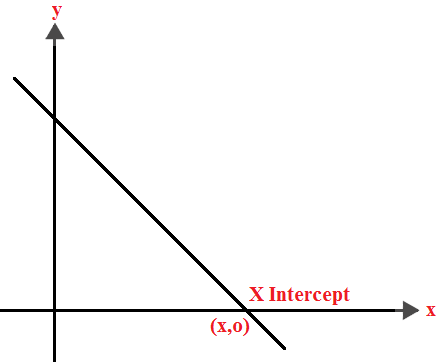Q

# I have a doubt, kindly clarify. - If a straight line passing through the point is such that its intercepted portion between the coord - Co-ordinate geometry - JEE Main

If a straight line passing through the point  is such that its intercepted portion between the coordinates axes is bisected at P, then its equation is :

• Option 1)

• Option 2)

• Option 3)

• Option 4)

Views

x-intercept -

The distance on the x-axis from the origin where the straight line cuts it.

- whereinIntercept form of a straight line -

- wherein

and are the -intercept and -intercept respectively.

So that line say intersect y-axis at (o,b ) and x-axis at (a,o).

since it is bisected at p (-3,4)

Option 1)

Option 2)

Option 3)

Option 4)

Exams
Articles
Questions